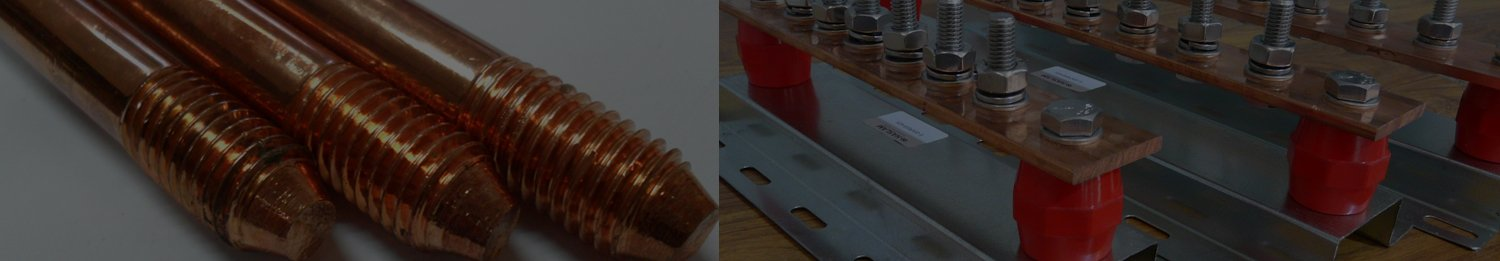# Copper Rod Weight Calculator

Results

To calculate the weight of a copper rod, you need to know the dimensions of the rod (length, diameter, or cross-sectional area) and the density of copper. The formula to calculate the weight (mass) of an object is:

Weight = Volume × Density

For a cylindrical rod like a copper rod, the formula for volume depends on its dimensions. There are two common cases: when you have the diameter and length, or when you have the cross-sectional area and length.

**Case 1: When you have the diameter and length of the rod:**

1. Find the volume of the cylinder:
Volume = π × (Diameter/2)^2 × Length

2. Find the weight (mass) using the formula:
Weight = Volume × Density

**Case 2: When you have the cross-sectional area and length of the rod:**

1. Find the volume of the cylinder:
Volume = Cross-sectional Area × Length

2. Find the weight (mass) using the formula:
Weight = Volume × Density

The density of copper is around 8.96 grams per cubic centimeter (g/cm³) or 8960 kilograms per cubic meter (kg/m³).

Here's an example calculation using Case 1:

Let's say you have a copper rod with a diameter of 2 cm and a length of 50 cm.

1. Volume = π × (2 cm / 2)^2 × 50 cm = 3.14 cm³

2. Weight = 3.14 cm³ × 8960 kg/m³ = 28,182.4 grams or 28.18 kilograms

And here's an example calculation using Case 2:

Let's say you have a copper rod with a cross-sectional area of 5 cm² and a length of 75 cm.

1. Volume = 5 cm² × 75 cm = 375 cm³

2. Weight = 375 cm³ × 8960 kg/m³ = 3,360,000 grams or 3360 kilograms

Remember to use consistent units in your calculations. In the above examples, I've used centimeters and grams for convenience. If you need the weight in other units, you can perform unit conversions as needed.